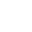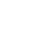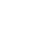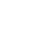Home » How I Taught Equivalent Fractions

# How I Taught Equivalent Fractions

Teaching equivalent fractions should use a variety of approaches, models and math tools.

Back when I was in high school, I drove home for lunch one day. We had just moved to a new city, though I continued to go to the same high school to finish my senior year. I took the freeway but this time, took a different exit. That exit turned into an hour-long drive because I was thoroughly LOST.

Lost as in I had no idea even which direction I was traveling! Remember, this was way before GPS – think Thomas Guide Maps! What does this have to do with equivalent fractions? After about 45 minutes of driving, the weather had cleared up (it had been very overcast). In front of me, I saw this very large hill. Bingo! Now I knew where I was. I saw a landmark that I recognized it it reoriented my direction and I was able to get home.

Understanding the benchmark fraction one-half is the same. When you understand half of anything, it helps you to find equivalents and compare other fractions. Is it more or less than one-half? Teaching the benchmark fraction one-half and its equivalents is the first step to comparing and finding equivalent fractions. Students need a guidepost!

I previously blogged about how I taught my third graders to compare fractions using bar models, number lines and fractions strips.  But what about equivalent fractions?  I used the same area models, number lines and fractions strips to teach equivalent fractions as hands-on concept building lessons. But then I added a new factor!  A Google Slides Interactive Digital Notebook!

## Where do Equivalent Fractions Fall in the Progression?

I recently came up with this chart after having done some further research into the teaching of fractions under the Common Core State Standards for Mathematics.  Most teachers believe that formal fraction teaching begins in third grade. But it doesn’t! It actually begins in kindergarten but falls under the geometry domain.

In geometry, students learn to partition shapes while beginning to learn the vocabulary of whole, halves, thirds, and fourths.  By second grade, students are introduced formally to the idea of equal parts.  Finally, in third grade, fractions are now taught as numbers.  Finding equivalent fractions falls near the end of the third-grade teaching cycle as students must first become familiar with comparing fractions as I explained in a related post.

## Hands-on to Find Equivalent Fractions

Up until second grade, students have been working on fractions at the concrete and representational levels. It’s not until third-grade that fractions become abstract numbers. But you still have to keep it hands-on at the concrete level to teach equivalent fractions!

My students were already familiar with using fraction strips since they had used them to compare fractions.  For equivalent fractions, I first let them form a complete fraction chart from one whole to 12 twelfths (though be aware that the Common Core State Standards for Math only deal with denominators of 2,3, 4, 6, and 8). They would use this math tool to now find equivalent fractions.

So I introduced a relatable situation. A would you rather have type situation.

Students could visually see that one-half was the same as three sixths. I instructed the students to pick up the 1/2 piece and find the fraction that is the same size (occupies the same space).  Was there anything else that was the same? Two-fourths, four-eighths, five-tenths, and six-twelfths!  We discussed the meaning of equivalent.

## What do you Notice about Equivalent Fractions?

I asked them to notice anything about the numerators and denominators. They could see that the denominator was double the numerator.  We had a discussion of why that is and came up with the idea that the numerator was HALF of the denominator.  BINGO!

That is why all these fractions are EQUIVALENT.   We continued to find equivalent fractions for fractions with denominators of thirds, fourths, sixths, and eighths.  This hands-on building of conceptual understanding of equivalent fractions should never be skipped or substituted.  Children DO need to manipulate solid, real objects in their hands and make these discoveries on their own.

Here is a video of one of my students hunting down an equivalent fraction using fraction strips.

We spent an entire lesson just using these manipulatives until I knew for sure the students understood the concept of equivalent fractions.  The next day my lesson focused on transferring that concept to number lines. I also introduced them to equivalent fraction charts with fraction strips and number lines as another math tool.  These happened to be included as a resource from the districted adopted math curriculum.

I started by demonstrating on the whiteboard how number lines could also be used to find equivalent fractions.  Then I had the students use their own whiteboards to draw the same size number lines and label them with halves and fourths.  We practiced several times until I could see that the class understood how to find equivalent fractions on a number line.  Now comes the exciting part!

## Using a Digital Notebook for Practicing Equivalent Fractions

My district is a 1:1 Chrome Book district in grades K – 8.  Each teacher has a cart with a Chrome Book for each student.  Many districts are opting for 1:1 to align instruction with the new online standardized tests known as SBAC and PARCC.  The technology component of these new standardized tests adds another challenge for students.

With that in mind, I designed my practice for the students using Google Slides.  I recently completed a major update to this resource to make it more adaptable for distance learning, hybrid-model learning or in-person learning. This resource now contains embedded tutorial videos! I created the videos myself to develop a conceptual understanding of equivalent fractions.

There are now slides that develop mathematical reasoning about fractions using routines, such as What do you Notice and Wonder? There are also many opportunities to practice building and finding equivalent fractions using the area model (circle fractions), length models (fraction strips), and fractions as number model (using the number line).

Mathematics is about developing reasoning skills to solve problems. The resource has many opportunities for students to problem-solve using equivalent fractions. Students reason while explaining their thinking about equivalent fractions..

The resource has 39 slides divided into 4 parts

• What are Equivalent Fractions?
• Finding Equivalent Fractions Using Lengths
• Finding Equivalent Fractions on a Number Line

If students are in a distance learning or hybrid model of learning, the embedded tutorial videos can be watched and rewatched to deepen the conceptual understanding of equivalent fractions. The teacher can also use the videos to integrate into synchronous live lesson.

Check out the full description and resource in my TpT Store!

Also, check out the  Comparing and Ordering Fractions.

## Don’t Go Yet!

Are you new to the 1:1 classroom setting? Then you’ll want to read my Valuable Tips for the 1:1 Classroom.

Check out how I use Google Classroom to present at Back to School Night for Parents.

Or register for the newsletter to receive this FREE Guide to Achieving Multiplication Fluency. Get it now by signing up for my newsletter below!###### Follow:

Hi, I’m Claudio, the dad from Two Boys and a Dad. Whether you’re just starting out teaching or a veteran teacher, let me offer you some ideas, tips, suggestions, resources and a sounding board for your daily classroom struggles (and successes!). This is your place to find information and ask questions about teaching in the elementary grades.

Share:### Interactive Digital Notebooks for Google Slides®